Multiplying 2-Digit x 1-Digit Numbers
Multiplying 3-Digit x 1-Digit Numbers
Multiplying 2-Digit x 2-Digit Numbers
Multiplying 3-Digit x 2-Digit Numbers
Mixed Word Problems
100

20 X 6 = ?

120

100

300 X 4 = ?

1,200

100

50 X 70 = ?

3,500

100

900 X 80 = ?

72,000

100

Is this an addition, subtraction, or multiplication word problem?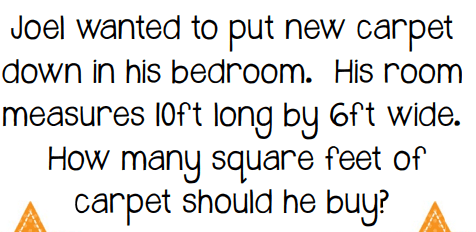It is a multiplication word problem. (This problem is asking you to find the area of the carpet in his bedroom. In order to find area of the carpet, you need to multiply length x width)

200

Solve the following multiplication problem:

21 x 4

84

200

Solve the following multiplication problem:

600 x 5

3,000

200

Solve the following multiplication problem:

900 x 60

54,000

200

Solve the following multiplication problem:

800 x 50

40,000

200

Is this an addition, subtraction, or multiplication word problem?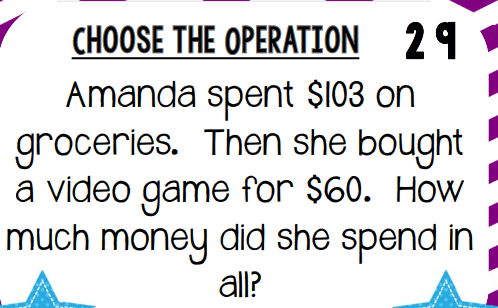This is an addition word problem. (You want to know how much money Amanda spent in all)

300

Solve the following multiplication problem:

35 x 9

315

300

Solve the following multiplication problem:

456 x 7

3,192

300

Solve the following multiplication problem:

99 x 27

2,673

300

Solve the following multiplication problem:

369 x 82

30,258

300

Is this an addition, subtraction, or multiplication word problem?It is a subtraction word problem. (You need to find the difference between how many days Melanie spent in school and how many days her sister spent in school)

400

Identify the error that was made.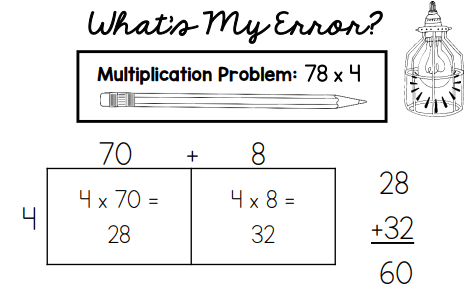Missing zero: 4 x 70 = 280

280

+ 32

312

400

Identify the error that was made.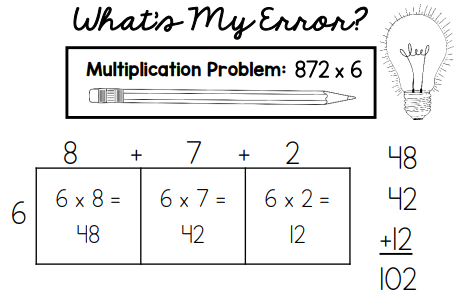Did not expand 872 correctly. It should have been 800 + 70 + 2.

6 x 800 =   4800

6 x 70   =     420

6 x 2      =  +  12

5,232

400

Identify the error that was made.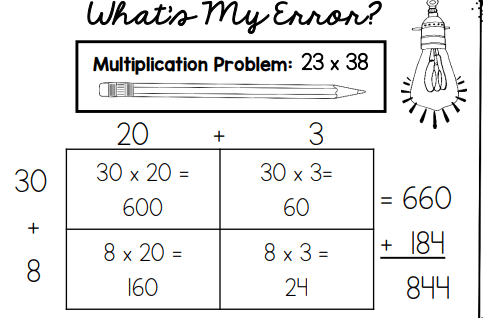They made a multiplication error in the 2nd box on the top. When they did 30 x 3, they added 3 + 3 and got 6. However, they needed to multiply 3 x 3 which would be 9 and then you would add a 0, making it to be 90 not 60.

600 + 90 =  690

160 + 24=   184

874

400

Identify the error that was made.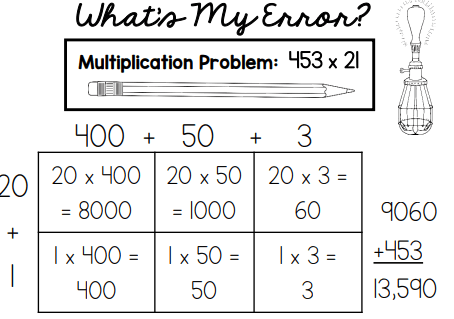It should have been:  9060

+  453

9513

400

Is this an addition, subtraction, or multiplication word problem?

There are 27 bananas in 9 equal bunches. How many bananas are in each bunch?

Division - you would have to divide 27 by 9 to find out how many bananas are in each bunch.

500

Alexis was reading her new book at a speed of 58 pages per night. If she reads every night for a week at this speed, how many pages would she have read?

*Think - How many days are in a week?*

After a week, Alexis would have read 406 pages.

500

The girl scouts are having their annual cookie sale. Emily sells 289 boxes of cookies each month. After 3 months, how many boxes of cookies did Emily sell?

After 3 months, Emily will have sold 867 boxes of cookies.

500

Mrs. O'Boyle bought each of the 36 kids in her class a pencil box. Each box has 12 pencils in it. How many pencils did she buy?

Mrs. O'Boyle bought 432 pencils.

500

Ava's garden has 138 rows of pumpkins. Each row has 24 pumpkins. How many pumpkins does she have in all?

Ava's garden has 3,312 pumpkins in all.

500

Solve the following word problem.

Daisy has 106 times more pears than Michelle. Michelle has 19 pears. The pears cost \$.68. How many pears does Daisy have?

Daisy has 2,014 pears.

Click to zoom